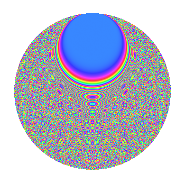# Properties

 Label 731.2.beLevel 731 Weight 2 Character orbit be Rep. character $$\chi_{731}(59,\cdot)$$ Character field $$\Q(\zeta_{56})$$ Dimension 1536 Newforms 1 Sturm bound 132 Trace bound 0

# Related objects

## Defining parameters

 Level: $$N$$ = $$731 = 17 \cdot 43$$ Weight: $$k$$ = $$2$$ Character orbit: $$[\chi]$$ = 731.be (of order $$56$$ and degree $$24$$) Character conductor: $$\operatorname{cond}(\chi)$$ = $$731$$ Character field: $$\Q(\zeta_{56})$$ Newforms: $$1$$ Sturm bound: $$132$$ Trace bound: $$0$$

## Dimensions

The following table gives the dimensions of various subspaces of $$M_{2}(731, [\chi])$$.

Total New Old
Modular forms 1632 1632 0
Cusp forms 1536 1536 0
Eisenstein series 96 96 0

## Trace form

 $$1536q - 20q^{2} - 20q^{3} - 28q^{5} - 48q^{6} - 48q^{7} - 36q^{8} - 44q^{9} + O(q^{10})$$ $$1536q - 20q^{2} - 20q^{3} - 28q^{5} - 48q^{6} - 48q^{7} - 36q^{8} - 44q^{9} - 20q^{10} - 20q^{11} - 20q^{12} - 52q^{14} - 20q^{15} + 192q^{16} - 20q^{17} - 64q^{18} - 20q^{19} - 52q^{20} - 20q^{22} - 16q^{23} - 12q^{25} - 4q^{26} - 44q^{27} + 28q^{28} - 20q^{29} - 4q^{31} - 36q^{32} + 32q^{33} - 52q^{34} - 8q^{35} - 120q^{36} - 16q^{37} - 44q^{39} - 108q^{40} - 36q^{41} - 48q^{42} - 76q^{43} + 48q^{44} - 100q^{45} - 100q^{46} - 132q^{48} - 88q^{49} - 320q^{50} - 20q^{51} - 40q^{52} - 24q^{53} + 60q^{54} + 88q^{56} + 52q^{57} + 36q^{58} - 44q^{59} + 36q^{60} + 16q^{61} + 84q^{62} - 44q^{63} - 60q^{65} + 76q^{66} + 120q^{67} - 52q^{68} - 88q^{69} - 4q^{70} - 68q^{71} - 40q^{73} - 252q^{74} + 120q^{75} - 208q^{76} - 100q^{77} + 204q^{78} + 80q^{79} + 32q^{80} - 140q^{82} - 96q^{83} - 104q^{84} + 192q^{85} - 24q^{86} - 88q^{87} + 128q^{88} - 52q^{90} + 200q^{91} + 592q^{92} - 400q^{93} - 188q^{94} - 84q^{95} + 88q^{96} - 60q^{97} + 112q^{99} + O(q^{100})$$

## Decomposition of $$S_{2}^{\mathrm{new}}(731, [\chi])$$ into irreducible Hecke orbits

Label Dim. $$A$$ Field CM Traces $q$-expansion
$$a_2$$ $$a_3$$ $$a_5$$ $$a_7$$
731.2.be.a $$1536$$ $$5.837$$ None $$-20$$ $$-20$$ $$-28$$ $$-48$$